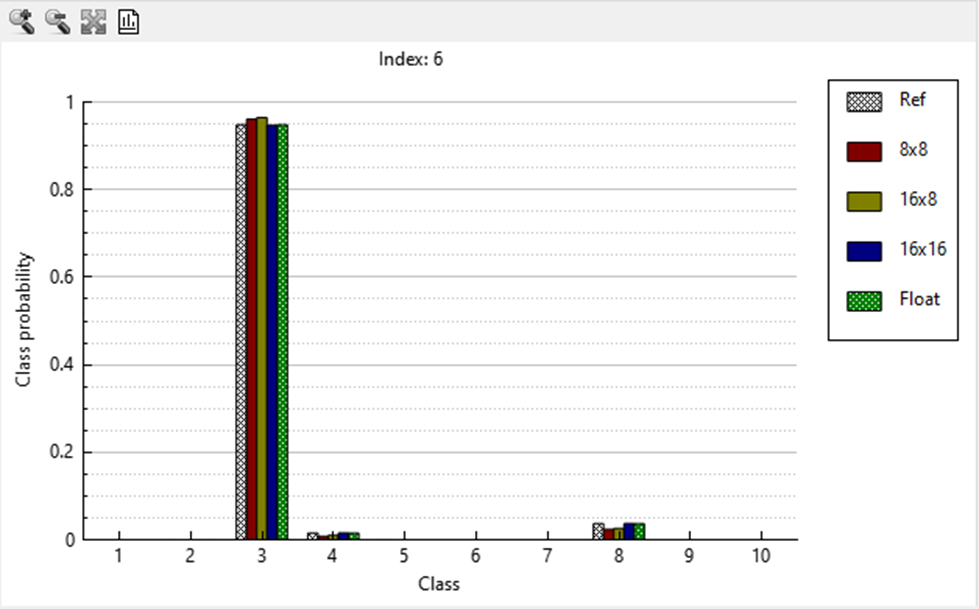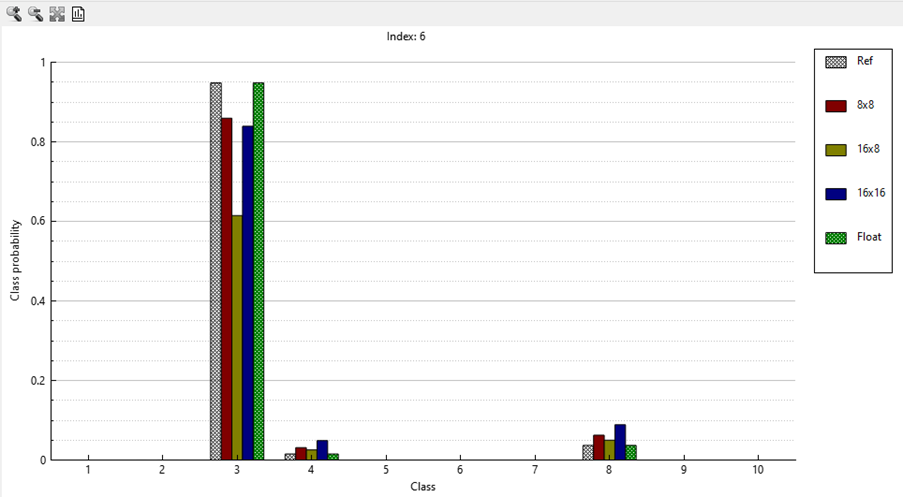# Change in probability while using ModusToolbox™ ML version 1.2 vs version 2.0 - KBA236672ModeratorModerator# Change in probability while using ModusToolbox™ ML version 1.2 vs version 2.0 - KBA236672

Community Translation: ModusToolbox™ ML version 1.2 とversion 2.0の違いにおける確率の変化 - KBA236672

Version: **

There is a change in probability when using the Infineon inference engine in ModusToolbox™ Machine Learning (ML) version 1.2 (Figure 1) compared to version 2.0 (Figure 2), with the same model and sample.Figure 1  Probability with ModusToolbox™ ML 1.2 inference engineFigure 2  Probability with ModusToolbox™ ML 2.0 inference engine

The change in probability is due to the difference in implementation of the softmax layer. In ModusToolbox™ ML version 1.2, the softmax layer was implemented using float operations, while in version 2.0, it is implemented using fixed-point operations. This change ensures that when an integer-based quantization is produced, all operations are integer-based, including the softmax layer.

The softmax layer is typically the last layer in a model and is used to normalize the output of the neural net into a probability distribution. When implementing the softmax layer with floating-point operations, the output probability will be more consistent with the original floating-point reference model than when using an integer-based softmax layer. This means that integer-based quantized model probabilities with version 2.0 will vary slightly when compared to version 1.2. The model will still perform as expected; the correct classification will be the one with the highest probability.

ModusToolbox™ ML 2.0 now includes support for the TensorFlow Lite for Microcontrollers (TFLM) inference engine, which supports float and int8x8 quantizations. The int8x8 quantized model for TFLM can be used in place of the integer-based Infineon quantized models.